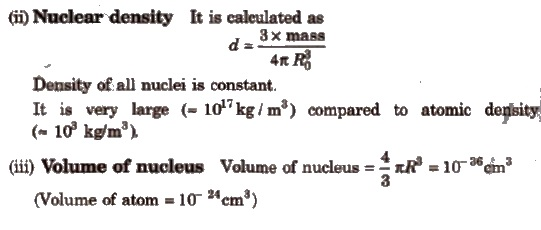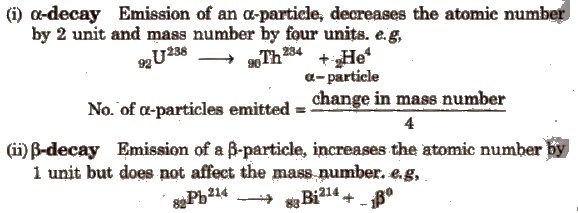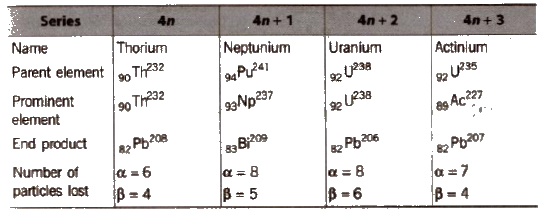The branch of chemistry which deals with the study of composition of atomic nucleus, nuclear forces, nuclear reactions and radioactive materials, is called nuclear chemistry.

Nucleons and Nuclear Forces

Protons and neutrons which reside in the nucleus, are called nucleons and forces binding them in the nucleus, are called nuclear forces.

These are short range forces operating over very small distances 1 fermi = 10– 15cm).

These forces are 1021 times stronger than the electrostatic forces.

no and p+ are held together by very rapid exchange of nuclear particles, called mesons (discovered by Yukawa). Mesons may be positively charged (π+), negatively charged (π) or neutral (πo).Parameter of Nucleus

(i) Radius of nucleus It is proportional to the cube root of the mass number of element.

R = Ro * A1 / 3

Where, A = mass number, R = radius of nucleus

Ro = proportionality constant = 1.4 x l0– 13 rn= 1.4 x 10– 15cm

Radius of the nucleus = 10– 13 – 10– 12cmFactors Affecting Stability of Nucleus

Stability of nucleus is affected by following factors

1.Neutron-proton Ratio or n / p Ratio

It is the main factor for determining the stability of nucleus.

The nuclei located in the zone of stability or belt of stability are stable.Nuclei lying above this zone, have higher n / p ratio and undergo β – emission to get the zone of stability.

Here, a no is transformed into p+ and give β and antineutrino. Thus, emission of β particles increases the number of proton and decreases the number of neutrons.2. Binding energy

It is the energy released when a nucleus is formed from its constituent nucleons.Higher the BE per nucleon, greater is the stability of the nucleus. Fe has maximum BE of 8.8 MeV per nucleon. Hence. it is most stable.

3. Packing fraction

It is the measure of relative mass defect.

Packing fraction = isotopic mass – mass no / mass no. * 104

Its value may be positive, negative or zero. Nuclei with positive packing fraction are highly unstable. Packing fraction is least for Fe and highest for H2.

4. Magic numbers

Nuclei having 2, 8, 20, 28, 50, 82 and 126 protons or neutrons are stable, hence these numbers are called magic numbers.

Nuclei with even numbers of both p+ and no are generally more stable than those with their odd numbers.

Certain nuclei spontaneously emit radiations. Such nuclei are called radioactive and this phenomenon of disintegration of nuclei spontaneously is called radioactivity.

It was discovered by Henri Becquerel in 1895. However, term radioactivity was given by Madam Curie.

Marie Curie and her husband Piere Curie isolated polonium and radium from pitchblende (UaOs)’

Radioactivity being a natural nuclear phenomenon, remains unaffected by external factors like temperature, pressure etc. Potassium uranyl sulphate [K (UO2) (SO4)2] was the first compound found to be radioactive. Tritium (1H ) is the lightest radioactive element.

Radioactive radiations are of three types : Positively charged α-rays, negatively charged β-rays and uncharged γ-rays.

Properties of α, β and γ-raysThe phenomenon of conversion of one radioactive nuclei into another with the emission of α, β and γ-rays is called radioactive disintegration.

It was proposed by Rutherford and Soddy in 1903.

γ-rays are secondary effects of radioactive disintegration i.e, emitted only after emission of α and β-rays.

Methods of Radioactive DisintegrationNo. of β -particles emitted = 2 x number of α -particles – (change in atomic number)

Emission of one α and two β-particles gives isotope.

(iii) γ-decay Emission of γ-rays does not affect the atomic number as well as mass number.

Group Displacement Law

This law was given by Soddy, Russel and Fajans in 1913 to decide the position of element, obtained after radioactive disintegration, in the Periodic Table.

According to this law, ‘In an a-emission, the daughter nuclei will occupy a position which is two places left to the position of parent nuclei and in a p-emission, the daughter nuclei will occupy a position one place right to the parent nuclei”.

Rate of DiSintegration

It depends only upon the number of atoms present and is defined as the number of atoms of radioactive element that disintegrate in a unit time.Half-life Period, t1/2

It is defined as the time required for one-half of the isotope to decay. It is calculated as

t1/2 = 0.693 / k

Its unit is t-1.

Half-life is related to total time as, T = n x t1/2Average Life (λ)

The statistical average of the lives of all atoms present at any time is called the ‘average life’. It has been shown to be reciprocal of decay constant, k.Activity of Radioactive Substance

Activity is defined as the number of disintegrations occurring in a radioactive substance per second.

Higher is the activity of a substance, faster will be its disintegration.Geiger Muller counter is used to determine the activity of a radioactive sample.

(i) Curie The activity of a substance, disintegrating with a rate of 3.7 x 1010 disintegration per second (dps), is said to be 1 curie.

1 Curie = 3.7 * 1010 dps

1 millicurie = 3.7 * 107 dps.

(ii) Rutherford It is the activity of a radioactive substance which disintegrates with a rate of 106 dps.

1 Rutherford (rd) = 106 dps.

(iii) Becquerel It is the activity of the substance, which disintegrates with a rate of 1dps. It is the 81unit of radioactivity.

1Bq (Becquerel) = 1 dps

Disintegration Series

These are the decay series in which heavy nuclei decay by a series of α and β emissions finally resulting in the formation of stable isotopes of

There are three natural disintegration series while the fourth series, called the neptunium series is artificialTo find a series, mass number is divided by 4.

The phenomenon of conversion of a stable nuclei into a radioactive nuclei by bombarding it with a high speed projectile is called artificial radioactivity or induced radioactivity (given by Curie and Joliot).

The element with atomic number greater than 92 (after U) are obtained by this process. These are called transuranium elements.

These all are synthetic and radioactiveArtificial Transmutation

The conversion of a non-radioactive element into another non-radioactive elements by artificial means, i.e, by bombarding with some fundamental particles, is called artificial transmutation. e.g,Nuclear Reactions

In these reactions, the nuclei of atoms interact with other nuclei or elementary particles such as α, β, p, d, n etc., and results in the formation of a new nucleus and one or more elementary particles.

A nuclear reaction can be represented as

parent nuclei (projectile, obtained particle) daughter nuclei

e.g, the reaction,Energy of a nuclear reaction, Q = (total mass of products – total mass of reactants) x 931.5 MeV.

For exoergic reactions, Q is negative and for endoergic reactions, Q is positive.

Types of Nuclear ReactionsAccelerators

These are used to give projectiles. Commonly used accelerators are linear accelerator, cyclotron and synchrotron.

Synchrotron is used as proton accelerator. Neutron being neutral are best projectile, protons and deuterons being monopositive are better projectile than u-particles (dipositive).

Nuclear Fission (0 Hahn and Fritz)

It is the process of splitting of a heavy nucleus into fragments with the emission of a large amount of energy. e.g,Nuclear fission is a chain reaction, e.g., it involves a series of reactions in which the product of the reaction initiate other similar reactions. e.g,During fission, there is always lose of mass which is converted to energy according to Einstein equation.

E = Δmc2,(where, Δm = mass defect)

The minimum mass of fissionable material required to sustain a chain reaction is called critical mass. If the mass of the fissionable material is more than the critical mass, it is called supercritical mass and if the mass of the fissionable material is smaller than the critical mass, it is called subcritical mass.

For U-235, the critical mass lies between 1 kg to 100 kg.

A nuclear fission process may be controlled or uncontrolled. Reactors are the examples of controlled chain reaction while atom bomb is an example of uncontrolled chain reaction.

Nuclear Reactor

In it, the nuclear fission process is carried out in a controlled manner. control rods made up of B or Cd absorb additional neutrons and thus, slow down the reaction. A nuclear reactor consists of :

(a) Fissile material U enriched in 92U235 is used as fissile material.
(b) Moderator These are used to slow down the speed of neutrons. e.g, graphite, heavy water, (D2O).
(c) Control rods Rods of B or Cd.
(d) Coolant Liquid alloy of Na and K is used as coolant. It takes away the heat of the exchanger. Heavy water, polyphenols and CO2 have also been used as coolants.

Breeder Reactor

This reactor also uses uranium as fuel but unlike a conventional reactor, it produces more fissionable materials than it uses.

U-235 or Pu-239 mixed with U-238 is used as a fuel in a typical Breeder reactor, so that breeding takes place within the core. Here, U-235 (or Pu-239) undergoes fission and U-238 capture more than one neutron (which are obtained during fission) to generate Pu-239. Thus, the amount of fissionable material can be steadily increased as the
starting nucleus fuels are consumed.

Fertile and fissile materials A fertile material is non-fissile in nature but can be made possible by reactions with neutrons. e.g, U238,Th232.

Fissile materials readily undergo fission and produce a large amount of energy. e.g,U235, Pu239 , U233 etc.

Atom Bomb

It contains two pieces of a fissile material having subcritical mass and an explosive. At the time of explosion, the explosive explodes and brings the subcritical masses together. Thus, a single substance having supercritical mass is obtained, which starts chain reaction and a huge amount of energy is released leading to explosion.

Nuclear Fusion

It is the process of conversion of two or more lighter nuclei into a heavier nuclei with the emission of a large amount of energy.

For the initiation of these reactions, a very high temperature (> 106K) is required so, these are also called thermonuclear reactions.

Energy of Sun and hydrogen bomb are based on the principle of nuclear fusion.

Following reactions are assumed as the source of energy of the Sun.Every second, the Sun loses 4.3 X 109 kg (430000 tons) of mass by the fusion process. But the total mass of the Sun is so great that this loss of mass is imperceptible.

Hydrogen Bomb

It contains a mixture of D2O and T2O (tritium oxide) surrounding an ordinary atomic bomb. The temperature produced by the explosion of the atomic bomb initiates the fusion reaction between 1H3 and 1H2

1. Estimation of Age (Dating Technique)

(i) Carbon dating technique It is used to determine the age of wood, animal fossils etc. It is based upon the ratio of C14 to C12 which remains constant in living organisms but decreases in dead sample. By comparing these two, the age is determined2. Medicinal Use

Radioisotopes are used to diagnose and cure many diseases. These can be used in three ways.

(i) In Vivo studies 51Cr is used for such technique.

(ii) In therapeutic procedure (to cure diseases) Co- 60 is used for the treatment of cancer, Na-24 is injected to trace the Dow of blood, 1-131 is used for the treatment of thyroid and P-32 is used for leukemia.

(iii) Imaging procedure 1-131 is used to study the structure and activity of thyroid gland. 1-123 is used in brain imaging and Tc-99 M is used in bone scans.

Radioisotopes are also widely used to find the reaction mechanism, in industry and in agriculture.